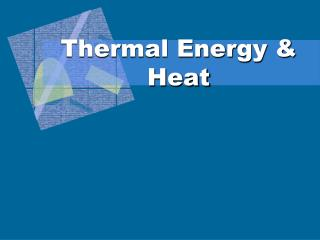DownloadDownload PresentationThermal Energy & Heat

# Thermal Energy & Heat

Download Presentation## Thermal Energy & Heat

- - - - - - - - - - - - - - - - - - - - - - - - - - - E N D - - - - - - - - - - - - - - - - - - - - - - - - - - -
##### Presentation Transcript

1. Thermal Energy & Heat

2. What is Temperature? • Temperature • measure of the averageKE of all the particles within an object

3. Temperature Conversions • oC to oF: oF = 9/5(oC) + 32 • oF = 1.8(oC) + 32 • oF to oC: oC = 5/9(oF – 32) • oC = 0.56(oF – 32) • oC to K: K = oC + 273 • K to oC: oC = K – 273

4. Going from Celsius to Fahrenheit Convert 37 oC to oF. oF = 9/5(oC) + 32 oF = 1.8(37oC) + 32 = 66.6 + 32 = 98.6oF

5. Going from Fahrenheit to Celsius Convert 68oF to oC oC = 5/9(oF – 32) oC = .56(68 – 32) = .56(36) = 20 oC

6. Going from Celsius to Kelvin Convert 100oC to K K = oC + 273 K = 100 + 273 = 373 K

7. Going from Kelvin to Celsius Convert 310 K to oC oC = K – 273 oC = 310 – 273 = 37oC

8. Thermal Energy • Thermal Energy • the total energy of the particles in a material • KE - movement of particles • PE - forces within or between particles due to position • depends on temperature, mass, and type of substance

9. 80ºC 80ºC 400 mL A B 200 mL Thermal Energy • Which beaker of water has more thermal energy? • B - same temperature, more mass

10. Heat Transfer • Heat • thermal energy that flows from a warmer material to a cooler material • Hot cold • Like work, heat is... • measured in joules (J) • a transfer of energy

11. 80ºC 10ºC A B Heat Transfer • Why does A feel hot and B feel cold? • Heat flows from A to your hand = hot. • Heat flows from your hand to B = cold.

12. Heat Transfer • Heat is always transferred from hot to cold. • Insulators slow the transfer of heat due to air pockets. • Conductors easily allow the transfer of heat, like metals. • Heat is transferred by conduction, convection, and radiation.

13. Conduction • Heat is transferred due to objects directly touching each other or through collisions. • Occurs best in solids. Heat continues to be transferred until both objects reach the same temperature, called a thermal equilibrium.

14. Convection • Transfer of heat through a liquid or gas through moving currents, called convection currents. • The cause of wind and weather.

15. Conduction vs Convection • http://energy.concord.org/energy2d/comparing-convection.html

16. Radiation • Transfer of heat through electromagnetic radiation (lightfrom stars or light bulbs). • Transferred in all directions. • No contact required! • Dark or dull objects absorb more than light or shiny objects do.

17. Heat Transfer • Specific Heat (Cp) • amount of energy required to raise the temp. of 1 kg of material by 1 degree Kelvin • units: J/(kg·K)or J/(g·°C)

18. 50 g Al 50 g Cu Heat Transfer • Which sample will take longer to heat to 100°C? • Al - It has a higher specific heat. • Al will also take longer to cool down.

19. – Q = heat loss + Q = heat gain T = Tf - Ti Heat Transfer Q = m T  Cp Q: heat (J) m: mass (g) T: change in temperature (K or °C) Cp: specific heat (J/g·K or J/g.oC)

20. Coffee cup Calorimeter Heat Transfer • Calorimeter • device used to measure changes in thermal energy • in an insulated system, heat gained = heat lost

21. Heat Transfer • A 32-g silver spoon cools from 60°C to 20°C. How much heat is lost by the spoon? GIVEN: m = 32 g Ti = 60°C Tf = 20°C Q = ? Cp = 235 J/kg·K WORK: Q = m·T·Cp m = 32 g = 0.032 kg T = 20°C - 60°C = – 40°C T = 293 K – 333 K = -40 K Q = (0.032kg)(-40 K)(235J/kg·K) Q = -300.8 J (lost heat, negative)

22. Heat Transfer • How much heat is required to warm 230 g of water from 12°C to 90°C? GIVEN: m = 230 g Ti = 12°C Tf = 90°C Q = ? Cp= 4.184 J/g·oC WORK: Q = m·T·Cp m = 230 g T = 90°C - 12°C = 78°C Q = (230 g)(78oC)(4.184 J/g·oC) Q = 75,061 J (gained heat, positive)